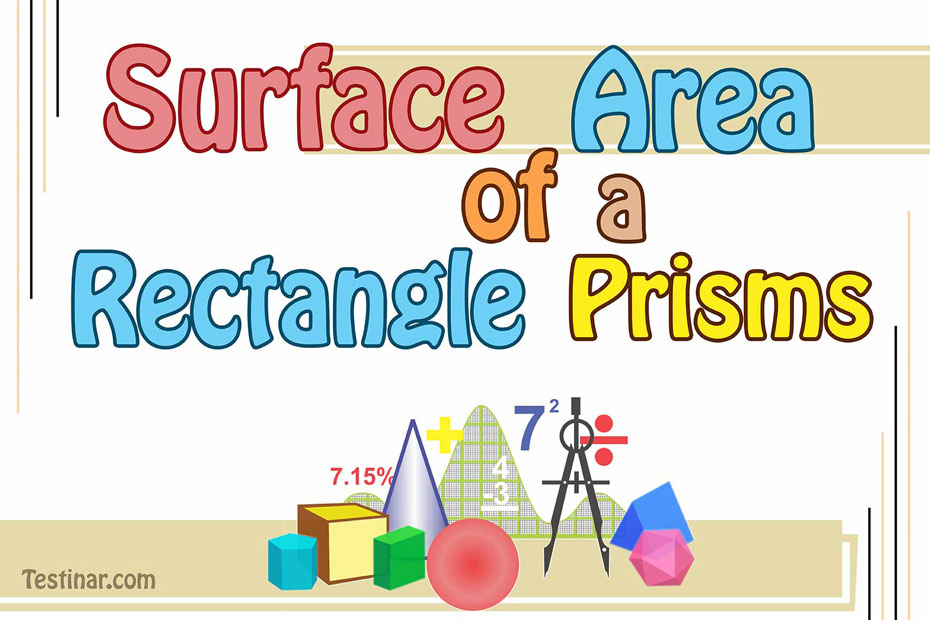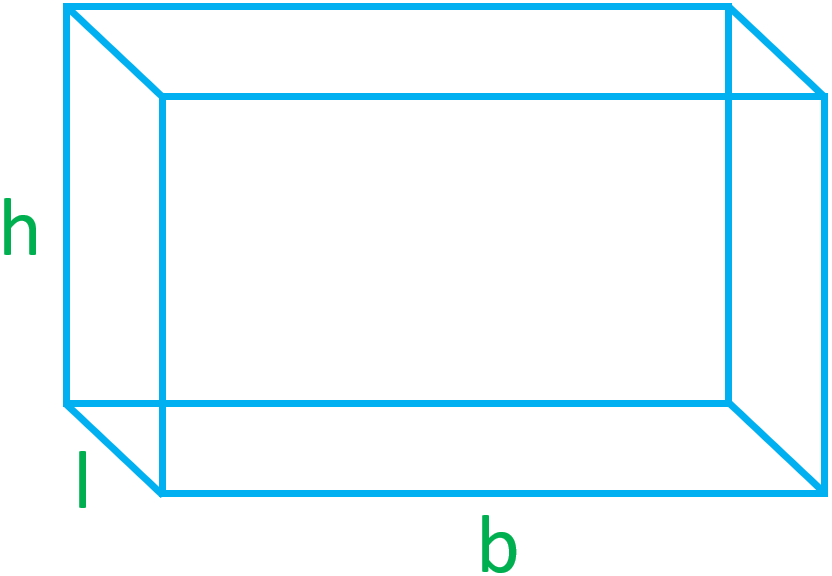## How to Find the Surface Area of a Rectangular Prism

A prism is a polyhedron having two bases that are parallel to one another. A rectangular prism has two similar rectangular bases and four rectangular faces. The rectangular prism's surface area equals the sum of the areas of its side faces and rectangular bases. Square units are used to measure the surface area of a rectangular prism.

Here is an example of a rectangular prism:The formula for the surface area of a rectangular prism is, $$2(bl \ + \ lh \ + \ hb)$$

Where,

• $$b$$ is the length of the base of the prism.
• $$l$$ is the width of the base of the prism.
• $$h$$ is the height of the square prism.

### Example:

Find the surface area of a prism with a base of $$6$$ cm, a height of $$14$$ cm, and a side of $$3$$ cm.

Solution:
Given, $$b \ = \ 6$$ cm, $$l \ = \ 3$$ cm, $$h \ = \ 14$$ cm
Surface area of rectangle prism $$= \ 2(bl \ + \ lh \ + \ hb) \ = \ 2(18 \ + \ 42 \ + \ 84) \ = \ 288$$ cm$$^2$$

### Exercises for Surface Area of a Rectangle Prism

1)2)3)4)5)6)7)8)9)10)1) $$1936$$ mm$$^2$$2) $$718$$ in$$^2$$3) $$88$$ cm$$^2$$4) $$808$$ m$$^2$$5) $$2086$$ cm$$^2$$6) $$2238$$ m$$^2$$7) $$94$$ m$$^2$$8) $$130$$ in$$^2$$9) $$1006$$ mm$$^2$$10) $$2398$$ in$$^2$$## Surface Area of a Rectangle Prism Practice Quiz

### SHSAT Mathematics Formulas

$6.99$6.99

### ASVAB Arithmetic Reasoning and Mathematics Knowledge Formulas

$9.99$5.99

### Accuplacer Math Full Study Guide

$25.99$12.99

### The Most Comprehensive ISEE Upper Level Math Preparation Bundle

$76.99$36.99